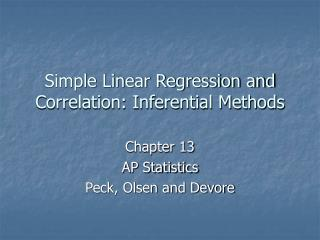DownloadDownload PresentationSimple Linear Regression and Correlation: Inferential Methods

# Simple Linear Regression and Correlation: Inferential Methods

Download Presentation## Simple Linear Regression and Correlation: Inferential Methods

- - - - - - - - - - - - - - - - - - - - - - - - - - - E N D - - - - - - - - - - - - - - - - - - - - - - - - - - -
##### Presentation Transcript

1. Simple Linear Regression and Correlation: Inferential Methods Chapter 13 AP Statistics Peck, Olsen and Devore

2. Topic 2: Summary of Bivariate Data • In Topic 2 we discussed summarizing bivariate data • Specifically we were interested in summarizing linear relationships between two measurable characteristics • We summarized these linear relationships by performing a linear regression using the method of least squares

3. Least Squares Regression • Graphically display the data in a scatterplot • Form, strength and direction • Calculate the Pearson’s Correlation Coefficient • The strength of the linear association • Perform the least squares regression • Inspect the residual plot • Determine if the model is appropriate • No patterns • Determine the Coefficient of Determination • How good is the model as a prediction tool • Use the model as a prediction tool

4. Interpretation • Pearson’s correlation coefficient • Coefficient of Determination • Variables in • Standard deviation of the residuals

5. Minitab Output

6. Simple Linear Regression Model • ‘Simple’ because we had only one independent variable • We interpreted as a predicted value of y given a specific value of x • When we can describe this as a deterministic model. That is, the value of y is completely determined by a given value x • That wasn’t really the case when we used our linear regressions. The value of y was equal to our predicted value +/- some amount. That is, We call this a probabilistic model. • So, without e, the (x,y) pairs (observed points) would fall on the regression line.

7. Now consider this … • How did we calculate the coefficients in our linear regression models? • We were actually estimating a population parameter using a sample. That is, the simple linear regression is an estimate for the population regression line • We can consider estimates for

8. Basic Assumptions for the Simple Linear Regression Model • The distribution of e at any particular value of x has a mean value of 0. That is, • The standard deviation of e is the same for any value of x. Always denoted by • The distribution of e at any value of x is normal • The random deviations are independent.

9. Another interpretation of • Consider , where the coefficients are fixed and e is distributed normally. Then the sum of a fixed number and a normally distributed variable is normally distributed (Chapter 7). So y is normally distributed. • Now the mean of y will be equal to plus the mean of e which is equal to 0 • So another interpretation is the mean y value for a given x value =

10. Distribution of y • Where we can now see that y is distributed normally with a mean of • The variance for y is the same as the variance of e -- which is • An estimate for is

11. Assumption • The major assumption to all this is that the random deviation e is normally distributed. • We’ll talk more about how this assumption is reasonable later.

12. Inferences about the slope of the population regression line • Now we are going to make some inferences about the slope of the regression line. Specifically, we’ll construct a confidence interval and then perform a hypothesis test – a model utility test for simple linear regression

13. Just to repeat … • We said the population regression model is • The coefficients of this model are fixed but unknown (parameters) – so using the method of least squares, we estimate these parameters using a sample of data (statistics) and we get

14. Sampling distribution of b • We use b as an estimate for the population coefficient in the simple regression model • b is therefore a statistic determined by a random sample and it has a sampling distribution

15. Sampling distribution of b • When the four assumptions of the linear regression model are met • The mean value of the sampling distribution of b is . That is, • The standard deviation of the statistic b is • The sampling distribution of b is normally distributed.

16. Estimates for … • The estimate for the standard deviation of b is • When we standardize b it has a t distribution with n-2 degrees of freedom

17. Confidence Interval • Sample Statistic +/- Crit Value * Std Dev of Stat

18. Hypothesis Test • We’re normally interested in the null because if we reject the null, the data suggests there is a useful linear relationship between our two variables • We call this ‘Model Utility Test for Simple Linear Regression’

19. Summary of the Test • Test Statistic • Assumptions are the same four as those for the simple linear regression model.

20. Minitab Output